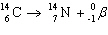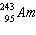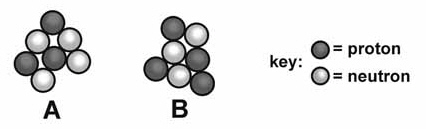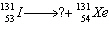Name:    Chemistry Chapter 4 Study Guide

Completion
Complete each statement.

1.

The concept that matter was composed of tiny indivisible particles was originally given by ____________________.

2.

Researchers discovered a subatomic particle while working with the cathode ray tube. The particle is known as a(n) ____________________.

3.

A ____________________ is a subatomic particle that has mass nearly equal to that of a proton, but it carries no electrical charge.

4.

Atoms with the same number of protons but different number of neutrons are called ____________________.

5.

Uranium is a naturally occurring element that emits particles and rays spontaneously through a process called _____________________.

6.

An alpha particle is _____________________ charged with two protons and neutrons.

7.

In the equation, the ___________________ decay of radioactive carbon-14 results in the creation of a new nitrogen-14 atom.

8.

A beta particle consists of a fast moving electron and has a(n) _____________________ charge.

9.

In nanotechnology, individual atoms are seen using the ____________________ microscope.

10.

The mass of an electron is ____________________ g.

11.

James Chadwick showed that the nucleus also contained a neutral subatomic particle known as the ____________________.

12.

Democritus believed that matter is made up of tiny individual particles known as a(n) ____________________.

13.

The number of protons in an atom is called the ____________________ of the element.

14.

Write the nuclear equation to describe the alpha decay of.

15.

Compare and contrast mass number and atomic mass.16.

What is the average atomic mass of this element?
 Isotope Mass (amu) Percent Abundance Phosphorus-29 29 5% Phosphorus-31 31 71% Phosphorus-32 32 24%

17.

What is the average atomic mass of this element?
 Isotope Mass (amu) Percent Abundance Silver-105 105 48% Silver-108 108 43% Silver-109 109 9%

18.

Write the chemical symbol for the isotope of cobalt with 37 neutrons.

19.

Identify the missing particle in the equation below. Justify your response.20.

Write the nuclear equation to describe the beta-decay of boron-12.

21.

Element X has an average atomic mass of 64.32 amu. If this element consists of only two isotopes, X-64 and X-65, which isotope is present in the greater abundance? Explain how you can tell.

22.

Define an atom.

23.

What do you understand by the term atomic mass unit (amu)?

24.

What is a nuclear reaction?

25.

How are atomic number and mass number denoted in the chemical symbol of the isotope of an element? Express the shortened notation for an isotope of element X with atomic number 92 and atomic mass 238.

26.

Identify the element containing 34 protons.

27.

What were the main flaws in Dalton’s atomic theory?

28.

Fast moving electrons travel through the empty space surrounding the nucleus. How are electrons held within the atom?